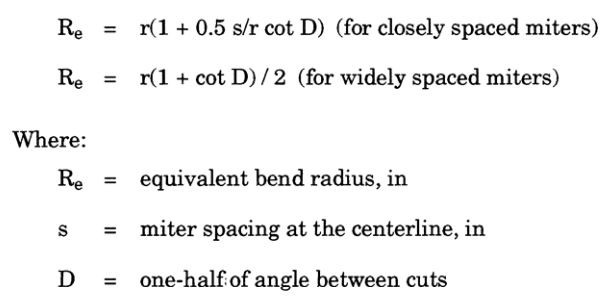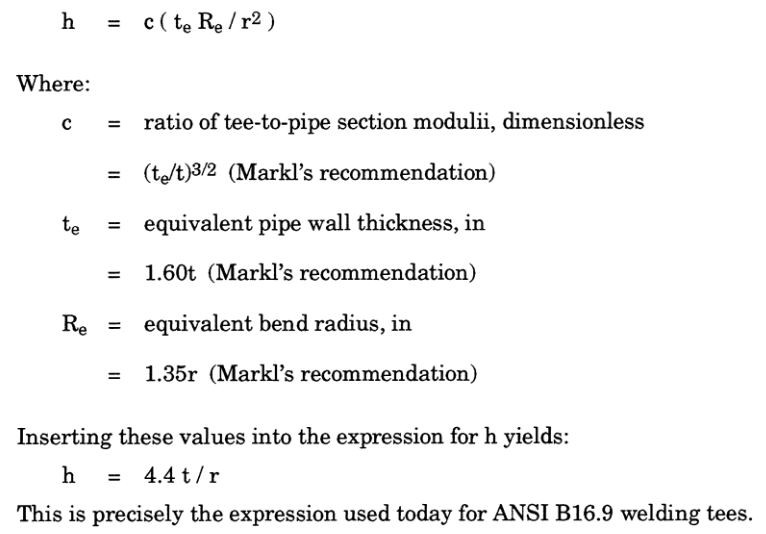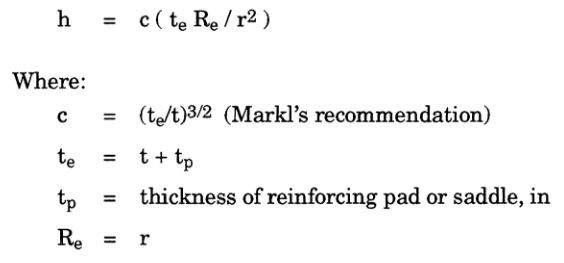Search

# 1.3 Stress Intensification Factors

As noted previously, Markl's fatigue tests generated endurance curves for various fitting configurations, such as straight pipe, butt welded pipe, elbows, miters, welding tees, unreinforced and reinforced fabricated tees, mostly using 4" nominal diameter, size-on-size fittings. Markl noticed that the fatigue failures occurred not in the middle of his test spans, but primarily in the vicinity of the fittings, and in those cases, they also occurred at lower stress/cycle combinations than for the straight pipe alone.

Earlier theoretical work pointed to a possible explanation. It had been shown that elbows tend to ovalize during bending, bringing the outer fibers closer to the neutral axis of the pipe, thus reducing the moment of inertia (increasing flexibility) and the section modulus (increasing developed stress).The stress intensification factors (the ratio of actual bending stress to the calculated bending stress for a moment applied to the nominal section) for elbows was known to be:Markl found this to correlate fairly well with his test data and so adopted it. Tests on mitered bends correlated well with those for smooth bends, providing an equivalent bend radius R was used in the above equation for h. Markl's estimates of equivalent bend radius are shown below:Markl found that the unreinforced fabricated tees could be modeled using the same formula as that for single (widely spaced) miter bends could be used, if a half angle of 45 degrees was used. This produces a flexibility characteristic of:For butt welded tees (such as ANSI B16.9 welding tees) Markl again adapted the bend equations, this time computing an equivalent radius (Re) and an equivalent thickness (te). Markl's equation for welding tees was:For reinforced fabricated tees, Markl used the expression he had previously used for welding tees, with different equivalent wall thickness and bend radius:The following tables compare the stress intensification factors suggested by Markl's test results versus the values calculated with his equations (results are for 4" nominal diameter, standard schedule pipe):These formulas for intensification factors were adopted (and expanded) by the piping codes. Specific formulas and/or fittings recognized by the individual ASME/ANSI B31 codes are usually shown in Appendix D of those codes (see Figure 1-23).Subsequent research has demonstrated that Markl's formulas, having been based on a limited number configurations (significantly having omitted reduced outlet tees) and disregarding any need to intensify torsional stress, are inaccurate in some respects.

The major problem with reduced intersections tees lies in the out-of-plane bending moment on the header. Stresses due to these moments can never be predicted from the extrapolation of size-on-size tests. Figure 1-24 below illustrates the origin of this problem.Errors due to these moments can be non-conservative by as much as a factor of two or three. Furthermore, when the r/R ratio is very small, the branch connection has little impact on the header, so use of large stress intensification factors for the header can produce unreasonably large calculated stresses.

R.W. Schneider of Bonney Forge pointed out this inconsistency for reduced branch connections.His paper on the subject states that the highest stress intensification factors occur when the ratio of the branch to header radii is about 0.7, at which point the nonconservativism (versus Markl's formulas) is on the order of two.